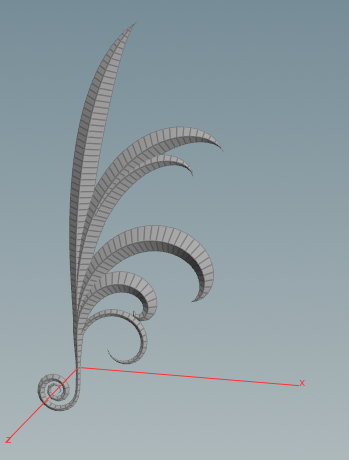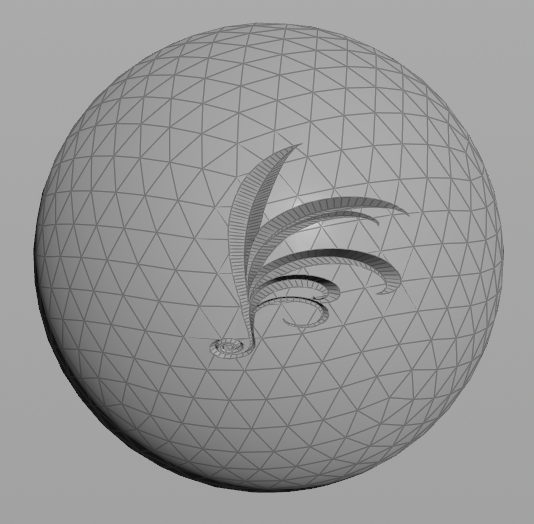## Recommended Posts

Hi Wizards

I'm trying to convert a poly shape to a NURBS surface or curve. So that i'm able to project that polyshape onto another object. So that I can trim it. The goal is make it look like the shape has been carved out of the other object.

Other solutions than projecting the NURBS surface are welcome too!Pattern on Ball.hipnc

##### Share on other sites

Hi Marco,

you can project and subtract geometry from meshes using uvsample() and boolean node.```float scale = chf('scale');
float scale_depth = chf('scale_depth');
vector2 offset = set(chf('offset_X'), chf('offset_Y'));
vector2 scale_uv = set(chf('scale_UV_X'), chf('scale_UV_Y'));

float depth = v@P.z;
vector bbox = relbbox(0, v@P);
vector size = getbbox_size(0);
float aspect = size.x / size.y;

vector lookup = bbox * aspect * scale * scale_uv + offset;

vector pos = uvsample(1, 'P', 'uv', lookup);
vector nml = uvsample(1, 'N', 'uv', lookup);

v@P = pos + nml * depth * scale_depth;```

Project_Mesh.hipnc

•3

##### Share on other sites
On 5-8-2019 at 6:53 PM, srletak said:

here you have solution

Thanks SreltakBut im not quite sure how to install and use this. Where do I drag the file and folders into?

On 6-8-2019 at 8:26 AM, konstantin magnus said:

Hi Marco,

you can project and subtract geometry from meshes using uvsample() and boolean node.

```
float scale = chf('scale');
float scale_depth = chf('scale_depth');
vector2 offset = set(chf('offset_X'), chf('offset_Y'));
vector2 scale_uv = set(chf('scale_UV_X'), chf('scale_UV_Y'));

float depth = v@P.z;
vector bbox = relbbox(0, v@P);
vector size = getbbox_size(0);
float aspect = size.x / size.y;

vector lookup = bbox * aspect * scale * scale_uv + offset;

vector pos = uvsample(1, 'P', 'uv', lookup);
vector nml = uvsample(1, 'N', 'uv', lookup);

v@P = pos + nml * depth * scale_depth;```

Oew, more new wrangle stuff. This works nicely.

Let me try figure out how this works. I'll write while I'm trying to understand this.

You create 6 float sliders with their names, respectively.

Another float called depth and connected it with the Positions in the Z axis. (If the to be projected model is in an other axis, edit this to the axis correspondingly
"vector bbox = relbbox(0, v@p);" Creating a vector called bbox, which is between 0 and vectors position attribute? in all axis?
"vector size = getbbox_size(0);" Creating a vector called size, which will get the bbox values of the just created vector 'bbox'? Given it a first thought It seems like this is double? as in, you've created a bbox before right? Not sure how this works.

"vector lookup = bbox * aspect * scale * scale_uv + offset;" This equation does the positioning on the object.

vector pos = uvsample(1, 'P', 'uv', lookup); By using the values from U and V, and 0,1 from the object you do the projection?
vector nml = uvsample(1, 'N', 'uv', lookup); Kinda like the same but with Normals.

"v@P = pos + nml * depth * scale_depth;"  This equation does the projection on the object? and set the depth of the carving.

Guess I understand partly what you're doing here. But hey the Vex works. I really appreciate your help!

Do you have by change some links or tuts of which I can learn more Vex and how to creatively use it.

## Create an account

Register a new account